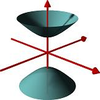#### You may also like### A Close Match

Can you massage the parameters of these curves to make them match as closely as possible?### Prime Counter

A short challenge concerning prime numbers.### The Right Volume

Can you rotate a curve to make a volume of 1?

# Googol

##### Age 16 to 18 Short Challenge Level:

The numbers in the problem are too large for computers to deal with in a straightforward fashion (or, rather, were in 2011), so we need to use pure mathematics to help us. There are at least two possible positive ways forwards:

First, you might notice that the inequality is a quadratic in the variable $n^2$. You could solve the corresponding equality and use this to work out the minimum value of $n$ by rounding up the answer to the next largest integer.

Alternatively, you could notice that $10^{100}=(10^{25})^4$. So, it is quite clear that $n=10^{25}$ is too small.

What about $10^{25}+1$? We can substitute this value and use the binomial theorem to show that $$\begin{eqnarray} (10^{25}+1)^4-6(10^{25}+1)^2&=&\left(10^{100} +4\times 10^{75} + 6\times 10^{50} +4\times 10^{25} + 1\right)\cr &&\quad\quad- 6\left(10^{50}+2\times 10^{25}+1\right) \cr &=& 10^{100}+4\times 10^{75}-8\times 10^{25}-5 \end{eqnarray}$$ It might seem 'obvious' that this value is greater than $10^{100}+1$ but it is a good idea to get into the habit of writing down precisely why it is obvious - the problem here is that we are suggesting the that sum of three different terms is greater than $1$. A simple way forward is to write out an inequality where we simplify one of the terms at each stage until we are left with just two terms as follows:
$$\begin{eqnarray} 4\times 10^{75}-8\times 10^{25}-5&> & 10^{75} - 8\times 10^{25}-5\cr &> & 10^{75}-10^{26}-5\cr &> & 10^{75}-2\times 10^{26}\cr &> & 10^{75}-10^{27}\cr &> &1 \end{eqnarray}$$
(Note: you might not see the 'point' of these inequality manipulations: they are useful because it is clear and easy to verify each individual step. This turns something which might just be controversial into something that is not at all controversial.)

Now for writing out the number $N$ on the left hand side of the inequality. As the number is so large a computer or a spreadsheet will not easily help us. Keeping the '+1' part separate for as long as possible gives us (where $X=10^{25}$)

$$\begin{eqnarray} N &=& (X+1)^4-6(X+1)^2 \cr &=& X^4+4X^3+6X^2+4X+1 - 6(X^2+2X+1)\cr &=& X^4+4X^3-8X-5 \end{eqnarray}$$ The structure of this number is now clear to us and includes the problematic negative terms, which we can deal with one at a time.

Firstly, $8\times 10^{25}$ is represented as $8$ followed by $25$ zeros. Removing this from the part $4\times 10^{75}$ leaves a number of the form

$$100\dots 0039\dots 9200\dots 00$$
Removing the $5$ from this gives a number of the form
$$100\dots 0039\dots 9199\dots 995$$
The trick is now to get the $1$s and the $3$ in the correct place. If we consider the number as a string of digits, counted from the right, then the first $1$ with be in the 101st place, the $3$ in the 76th place and the second 1 in the 26th place.

10,000,000,000,000,000,000,000,003,999,999,999,999,999,999,999,999,999,999,999,999,999,999,999,999,919,999,999,999,999,999,999,999,995

Phew! Who would have thought that place value could be so tricky? If you are planning on entering a career in finance or science then part of your computer programming will be to ensure that large numbers that you enter into your code are accurate. A lot could rest on this accuracy, so patient and careful detail are the key skills required.